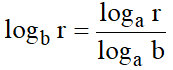# INFINITE SERIES

### BINOMIAL THEOREM FOR ANY INDEX

If | x | < 1, then for any nThe following are some important properties of this expansion:
1. In this expansions there are infinitely many terms.
2. This expansion is valid for | x | < 1 and first term unity.
3. The general term of this expansion is4. When x is small compared with 1, we see that the terms finally get smaller and smaller. If x is very small compared with 1, we take 1 as a first approximation to the value of (1 + x)n or 1 + nx as a second approximation.
5. Replacing n by –n in the above expansion, we getReplacing x by –x in this expansion, we getHence,### EXPONENTIALS

The number e
By the Binomial Theorem, if  n > 1,=If n is indefinitely large, thenetc. Then the right hand side =Thus, the limiting value ofwhen n is infinite, is the sum of the seriesThe sum of this series is denoted by the letter 'e'. Hence, we have,RESULTS :
• e is an irrational number.
• 2 < e < 3. The approximate value of e is e = 2.71828.

### EXPONENTIAL SERIES

To prove thatIf n is natural number greater than 1, then for any x,orTaking limit as, we getTo expand ax in ascending powers of x
We haveLet ec = a so that c = loge a.
Then ax = ec x =orThis is called the exponential theorem.

SOME IMPORTANT FORMULAE
1.2.3.4.5.6.7.8.Note :
(i)(ii)### LOGARITHMS (DEFINITION)

For each positive real number, the unique real number m is called the logarithm of b to the base a written as logab, if am = b.
• Thus logab = m, if and only if am = b, 'log' being the abbreviation of the word 'logarithm'.
• The following properties of logarithm follow immediately from the definition :
loga 1 = 0 because a0 = 1
loga a = 1 because a1 = a
loga ax = x because ax = ax
• Moreover, as a is a positive real number, b is also a positive real number. Thus, we define the logarithm of only a positive real number to a positive real number base other than 1. Negative numbers and zero have no logarithms. The expressions log (–2), log 0,are meaningless.

### LAWS OF LOGARITHMS

If a > 0 and , then
1.2.3.4. Change of base : For any positive real numbers r and b,,
5.6.7.8.for any positive x and c not equal to 1.
9.10.11.12.### LOGARITHMIC SERIES

If a is positive, then by the exponential theorem, we have
ay = 1 + y loge a +Putting a = 1 + x, we getIf | x | < 1, then by Binomial theorem, we have=+ terms involving higher powers of y
Thus,This is an identity in y, therefore, equating coefficients of y on both sides we getThis is known as the Logarithmic Series.

SOME IMPORTANT RESULTS
1.2.On Subtracting (2) from (1), we get
1.(–1 < x < 1)
2.### CALCULATION OF LOGARITHMS

We have...(1)
Suppose we have to find loge2. Putting, we getOn puttingin (1), we get
Similarly, we can findand so on.
Logarithms of numbers calculated to the base e are called NAPERIAN LOGARITHMS or NATURAL LOGARITHMS.
Logarithms to the base 10 are called COMMON LOGARITHMS.orHence, the logarithms of any number to the base 10 can be obtained by multiplying the logarithms of the number to the base e by 0.43429448.
Also we have 2 < e < 3
And logex is an increasing function, therefore log 2 < loge e < log 3  log 2 < 1 < log 3.

Example :
Prove thatSolution :
We have,## Want to know more

Please fill in the details below:

## Latest NEET Articles\$type=three\$c=3\$author=hide\$comment=hide\$rm=hide\$date=hide\$snippet=hide

Name

ltr
item
BEST NEET COACHING CENTER | BEST IIT JEE COACHING INSTITUTE | BEST NEET & IIT JEE COACHING: Infinite Series | Mathematics Notes for IITJEE Main
Infinite Series | Mathematics Notes for IITJEE Main